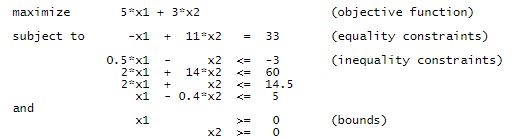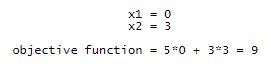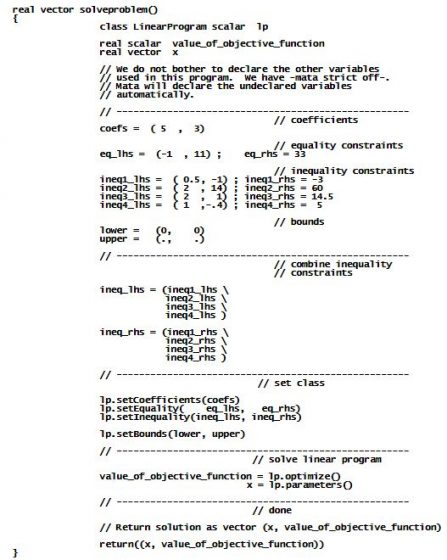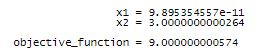Stata provides statistical solutions developed by StataCorp, and it provides programming tools for those who want to develop their own solutions. There are two Stata programming languages: ado, which is easy to use, and Mata, which performs numerical heavy lifting. And Stata is integrated with Python.

New Mata class LinearProgram() solves linear programs. It uses Mehrotra’s (1992) interior-point method, which is faster for large problems than the traditional simplex method.

Let’s see it work

Here’s a linear program that we will solve:The mathematical solution to this toy problem isLet’s use LinearProgram() to solve it. To do that, you

set the coefficients of the objective function,

set the equality constraints (if any),

set the inequality constraints (if any), and

set the bounds.

A program to fit the above model might read:Running this program results in